# Basic array techniques

ColdFusion arrays are a fundamental part of writing programs in ColdFusion. An array is simply an ordered stack of data items with the same data type. Using an array, you can store multiple values under a single name. Instead of using a separate variable for each item, you can use one array to hold all of them.

# Create an array

In ColdFusion, you can create arrays explicitly, by using a function to declare the array and then assigning it data, or implicitly by using an assignment statement. You can create simple or complex, multidimensional arrays.

To create an array explicitly, you use the ArrayNew function and specify the array dimensions, as in the following example:

`myArray=ArrayNew(2)`

This statement creates a two-dimensional array named myArray. You use this method to create an array with up to three dimensions.

After you create an array, you can add array elements, which you can then reference by using the element indexes.

For example, you can create a one-dimensional array called firstName:

`firstName=ArrayNew(1)`

The array firstName does not hold any data. When you add data into the array, as shown below:

```firstName=”John”
firstName=”Jason”```

The array has a length of three. When you dump the array contents, you get the following output: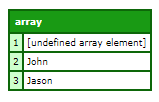You can also create arrays implicitly. To create an array implicitly, you use a new variable name on the left side of an assignment statement, and an array notation on the right side of the statement, as in the following example:

`firstNameImplicit=[“John”,”Jason”,”James”]`

This single statement is equivalent to the four statements used to create the firstname array in Creating arrays using functions.

When you create an array implicitly, the right side of the assignment statement has square brackets ([]) surrounding the array contents and commas separating the individual array elements. The elements can be literal values, such as the strings in the example, variables, or expressions. If you specify variables, do not place the variable names in quotation marks.

You can create an empty array implicitly, as in the following example:

`myArray=[]`

You can also create an array implicitly by assigning a single entry, as the following example shows:

`myArray=”ColdFusion”`

In ColdFusion, you can also allow nested implicit creation of arrays, structures, or arrays and structures. For example,

`jazzmen = [["Coleman","Charlie"],["Hawkins", "Parker"]]`

To create a two-dimensional array, for example, use a format such as the following:

```cp = ["Charlie", "Parker"]
dg = ["Dexter", "Gordon"]```

Tying them all, you have the following code:

```<cfscript>
cp = ["Charlie", "Parker"]
dg = ["Dexter", "Gordon"]
jazzmen = [["Coleman","Charlie"],["Hawkins", "Parker"]]
players=[cp,dg,jazzmen]
writeDump(players)
</cfscript>
```

Output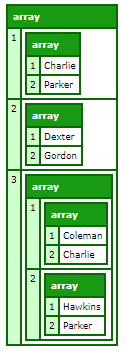# Create a multi-dimensional array

ColdFusion supports dynamic multidimensional arrays. When you declare an array with the ArrayNew function, you specify the number of dimensions. You can create an asymmetrical array or increase the number of dimensions by nesting arrays as array elements.

It is important to know that when you assign one array (array1) to an element of another array (array2), array1 is copied into array2. The original copy of array1 still exists, independent of array2. You can then change the contents of the two arrays independently.

The best way to understand an asymmetrical array is by looking at it. The following example creates an asymmetric, multidimensional, array, and the cfdump tag displays the resulting array structure. Several array elements do not yet contain data.

```<cfscript>
myotherarray=ArrayNew(2);
biggerarray=ArrayNew(3);
myarray=[];
biggerarray=myarray;
biggerarray=3;
biggerarray=myotherarray;
biggerarray="five deep";
biggestarray=ArrayNew(3);
biggestarray=biggerarray;
biggestarray="This is complex";
myarray="Can you see me";
writeDump(biggestarray);
writeDump(myarray);
</cfscript>
```

Output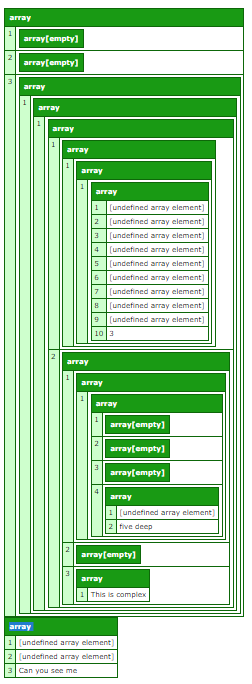Deconstructing the code, we have:

1. Create three empty arrays, a 2D, 3D, and a 1D array respectively.

```myotherarray=ArrayNew(2);
biggerarray=ArrayNew(3);
myarray=[]```

2. Make element  of the 3D array a copy of the 1D array.

`biggerarray=myarray;`

3. Assign 3 to the  element of the resulting array. The biggerarray array is now asymmetric. For example, it does not have a  element.

`biggerarray=3;`

4. Make element  of the 3D array be the 2D array.

`biggerarray=myotherarray;`

5. Assign the  element the value "five deep". The biggerarray array is now even more asymmetric.

`biggerarray="five deep";`

6. Create a second 3D array.

`biggestarray=ArrayNew(3);`

7. Make the element in  of this array a copy of the biggerarray array and assign an element to . The resulting array is complex and asymmetric.

`biggestarray="This is complex";`

8. Assign a value to element 3 of myarray.

`myarray="Can you see me";`

9. Use writeDump to view the structure of biggestarray and myarray. Notice that the "Can you see me" entry appears in myarray, but not in biggestarray, because biggestarray has a copy of the original myarray values and the change to myarray does not affect it.

`writeDump(biggestarray);`

# Add elements to an array

You can use the following array functions to add data to an array:

### ArrayAppend

The ArrayAppend function an array element to an array.  Concatenates arrays when the merge argument is set to true and the value argument is an array.

For example,

```<cfscript>
myArray=[1,3,5,7,9]
toAppend=ArrayAppend(myArray,11,true)
writeOutput("Is the value appended successfully?" & toAppend & "<br/>")
writeOutput("The modified array is:")
writeDump(myArray)
</cfscript>
```

Output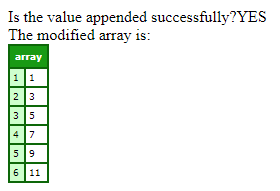### ArrayPrepend

The ArrayPrepend function inserts an array element at the beginning of an array.

For example,

```<cfscript>
myArray=[3,5,7,9,11]
toPrepend=ArrayPrepend(myArray,1)
writeOutput("Is the value pre-pended successfully?" & toPrepend & "<br/>")
writeOutput("The modified array is:")
writeDump(myArray)
</cfscript>```

Output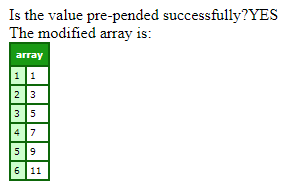### ArrayInsertAt

The ArrayInsertAt function inserts a value into an array. Array elements whose indexes are equal to or greater than the new position is incremented by one.

For example,

```<cfscript>
myCities=["London","New York","Paris","Tokyo","Barcelona"];
myNewCity="Berlin,Prague,Rome"
ArrayInsertAt(myCities,4,myNewCity)
writeOutput("The updated array is:")
writeDump(myCities)
</cfscript>```

Output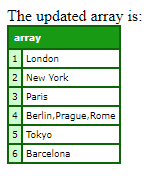# Delete elements in an array

You can use the following functions to delete elements from an array.

### ArrayDelete

The ArrayDelete function searches an array for the first position of a specified object and deletes it.

For example,

```<cfscript>
myArray=["London", "New York", "Paris", "Barcelona", "Berlin", "Tokyo", "Seattle"];
ArrayDelete(myArray,"Paris")
writeOutput("The modified array is:")
writeDump(myArray)
</cfscript>```

Output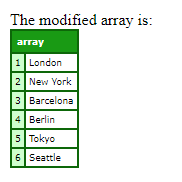### ArrayDeleteAt

The ArrayDeleteAt function deletes an element from a specified position in an array.

For example,

```<cfscript>
myArray=["London", "New York", "Paris", "Barcelona", "Berlin", "Tokyo", "Seattle"];
// Delete element at 4th position
ArrayDeleteAt(myArray,4)
writeOutput("The modified array is:")
writeDump(myArray)
</cfscript>```

Output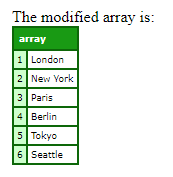# Loop over arrays

You can use different constructs for looping over arrays:

• for loops
• cfloop tag
• ArrayEach closures

### For loop

You can use for loops, as shown below:

```<cfscript>
myCities=["London", "New York", "Paris", "Barcelona", "Berlin", "Tokyo", "Seattle"];
// for loop
for (i=1;i<=ArrayLen(myCities);i++){
writeOutput("The city is:" & myCities[i] & "<br/>")
}
</cfscript>```

Output

The city is:London

The city is:New York

The city is:Paris

The city is:Barcelona

The city is:Berlin

The city is:Tokyo

The city is:Seattle

### cfloop tag

You can use for constructs, as shown below:

```<cfset myArray = ["Australia", "Brazil", "Canada"]>
<!--- By index --->
<cfloop index="i" from="1" to="#arrayLen(myArray)#">
<cfoutput>#myArray[i]#</cfoutput>
</cfloop>
<br/>
<!--- By array --->
<cfloop index="currentIndex" array="#myArray#">
<cfoutput>#currentIndex#</cfoutput>
</cfloop>```

Output

### ArrayEach

You can use ArrayEach, as shown below:

```<cfscript>
myCities=["London","New York","Paris","Tokyo","Barcelona"];
// Create a function that takes city as an argument  and prints the name of the cities as output
// with delimiter as space
ArrayEach(myCities,function(city){
WriteOutput("City is: " & city & "<br/>");
}
);
</cfscript>```

Output

City is: London

City is: New York

City is: Paris

City is: Tokyo

City is: Barcelona

# Functional programming with arrays

Using functional programming techniques, you can write code that is relatively bug-free, easier to debug and test, and easier to refactor.

One of the reasons why functional programming has become popular is its ability to manipulate data structures easily and efficiently when compared to traditional methods.

### ArrayMap

The ArrayMap function operates on all elements of an array and produces an array of the same dimension with transformed values.

For example,

```<cfscript>
myArray=[1,2,3,4,5]
ArrayMap(myArray,function(x){
doubled[x]=x*2 // each value of the original array is doubled by the closure function
})
writeDUmp(doubled)
</cfscript>```

Output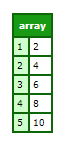### ArrayReduce

The ArrayReduce function “reduces” an array to a single value.

For example,

```<cfscript>
data = [1,2,3,4,5,6];
sum=data.reduce(function(previous,next) {
return previous+next;
},0);
writeOutput(sum); // Output is 21
</cfscript>```

Let’s deconstruct the code- The reduce function takes a callback function and an initial value as parameters. The callback function uses the parameters previous and next to add a current array element to its preceding element. When the function reaches the end of the array, the output is the sum of all elements in the input array.

### ArrayFilter

The ArrayFilter function filters the elements in an array for which the inline function returns true.

For example,

```<cfscript>
myArray=[1,2,3,4,5,6,7,8,9]
evenArray=ArrayFilter(myArray,function(item){
return (item mod 2==0)
})
writeOutput("The array of even numbers is:")
writeDump(evenArray)
</cfscript>```

Output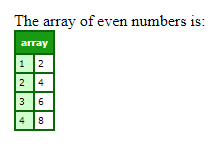# Other array functions

## Copy an array

You can copy arrays of simple variables (numbers, strings, Boolean values, and date-time values) by assigning the original array to a new variable name.

When you assign the existing array to a new variable, ColdFusion creates an array and copies the contents of the old array to the new array. The following example creates and populates a two-element array. It then copies the original array, changes one element of the copied array and dumps both arrays. As you can see, the original array is unchanged and the copy has a new second element.

For example,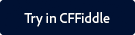```<cfscript>
myArray="First Array Element";
myArray="Second Array Element";
newArray=myArray;
newArray="New Array Element 2";
writeDump(myArray);
writeDump(newArray);
</cfscript>```

### Output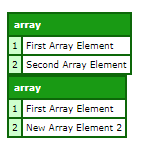## Length of an array

The function ArrayLen calculates the number of elements in an array.

For example,

```<cfscript>
myArray=[1,2,3,4,5];
writeoutput(ArrayLen(myArray)); // Returns 5
</cfscript>```

### More like thisKirjaudu sisään tiliisi